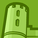# QlikView App Dev

Discussion Board for collaboration related to QlikView App Development.

Announcements
Action-Packed Learning Awaits! QlikWorld 2023. April 17 - 20 in Las Vegas: REGISTER NOW
cancel
Showing results for
Did you mean:Partner - Contributor III

## Variable in Class function

I am using the Class function to generate bucket ranges dynamically and having trouble getting the buckets to generate when inserting variables into the function.

Here is the Class function:  =Class(x, class_width, format)

Here is how I have tried to recreate it:  =Class(\$(vBucketRange), \$(vBucketBin), ' \$ ')

Can x be replaced with a formula or variable?

Any assistance would be greatly appreciated.

DETAILS:

1. In my requirement, x should be determined based on the larger value of two different fields . . .

x

=if(Flag_A=1 and Flag_B=1 and (PriceField1 > max(PriceField2)), PriceField1,

if(Flag_A=1 and Flag_B=1 and (PriceField1 < max(PriceField2)), max(PriceField2))

)

2. The class width should be the difference between the maximum value of two different fields and the minimum value of the same two different fields, divided by the number of bucket ranges selected by the user. . .

class_width =

=(if(max(PriceField1) > max(PriceField2), max(PriceField1), max(PriceField2

-
if(min(PriceField1) < min(PriceField2), min(PriceField1), min(PriceField2))

)
/
vNumberOfBuckets

I have created variables for the expressions above. Each variable renders the appropriate values when inserted into chart expressions. However, neither the variables nor the expressions seem to work within the Class function dimension.

1 Solution

Accepted SolutionsMVP

I am not sure how you are calculating the Bucket... But can try to help you out..

I will provide you how to find out the Max Price and Min Price...

Max Price

IF(Max(TOTAL <MyProduct> PriceField1) > Max(Total <MyProduct> PriceField2),

Max(TOTAL <MyProduct> PriceField1),

Max(Total <MyProduct> PriceField2))

Min Price

IF(Min(TOTAL <MyProduct> PriceField1) < Min(Total <MyProduct> PriceField2),

Min(TOTAL <MyProduct> PriceField1),

Min(Total <MyProduct> PriceField2))

Max Price - Min Price

IF(Max(TOTAL <MyProduct> PriceField1) > Max(Total <MyProduct> PriceField2),

Max(TOTAL <MyProduct> PriceField1),

Max(Total <MyProduct> PriceField2))

-

IF(Min(TOTAL <MyProduct> PriceField1) < Min(Total <MyProduct> PriceField2),

Min(TOTAL <MyProduct> PriceField1),

Min(Total <MyProduct> PriceField2))

Let me know if this would help !!

Otherwise, we can have another try with little more explanation from your end!

4 RepliesMVP

You are using max(PriceField1)... Try to use max(TOTAL PriceField1) for both PriceField1 and PriceFiel2. If still not work, kindly provide sample data..Partner - Contributor III
Author

Thank you for your response. I cannot provide an example of the app, but I have outlined the type of data I am working with - and an explanation of what I am trying to accomplish - in the table below. Based on the requirement, I am not sure that the Total() function will work. Please let me know if this provides enough additional insight.

 MyProduct Store CompetitorProducts PriceField1 (for My product) PriceField2 (for Comp products) Max Price of either PriceField1 or PriceField2 (based on price of my product and respective competitive products, in multiple stores).  In this example, PriceField1 should be used to replace 'x' in the Class function. Further, I would like the bucket ranges to be calculated based on the range of the highest price across the 2 price fields and the lowest. In this case, the high price is in PriceField1, and the low price is in PriceField2. Therefore, I want my range to be based on the following: Product_A Store1 CompProd_A \$14.99 \$14.99 \$16.99 \$14.79 - \$16.99 CompProd_B not priced CompProd_C \$15.40 CompProd_D price not available CompProd_E \$14.79 Product_A Store2 CompProd_A \$16.99 not sold in this store CompProd_B price not available CompProd_C \$14.99 CompProd_D store not evaluated CompProd_E \$14.99MVP

I am not sure how you are calculating the Bucket... But can try to help you out..

I will provide you how to find out the Max Price and Min Price...

Max Price

IF(Max(TOTAL <MyProduct> PriceField1) > Max(Total <MyProduct> PriceField2),

Max(TOTAL <MyProduct> PriceField1),

Max(Total <MyProduct> PriceField2))

Min Price

IF(Min(TOTAL <MyProduct> PriceField1) < Min(Total <MyProduct> PriceField2),

Min(TOTAL <MyProduct> PriceField1),

Min(Total <MyProduct> PriceField2))

Max Price - Min Price

IF(Max(TOTAL <MyProduct> PriceField1) > Max(Total <MyProduct> PriceField2),

Max(TOTAL <MyProduct> PriceField1),

Max(Total <MyProduct> PriceField2))

-

IF(Min(TOTAL <MyProduct> PriceField1) < Min(Total <MyProduct> PriceField2),

Min(TOTAL <MyProduct> PriceField1),

Min(Total <MyProduct> PriceField2))

Let me know if this would help !!

Otherwise, we can have another try with little more explanation from your end!Partner - Contributor III
Author

FINAL SOLUTION DETAILS:

Qlik’s popup explanation in dimension’s expression box: str Class(x, class_width[, forma[, bias]])

My Calculated Dimension expression: =class(\$(vBucketPriceField), \$(vBucketBin), '\$', \$(vBucketOffset))

Where the variables are defined as . . .

vBucketPriceField: To determine which field to use based on highest value between the two fields.

if(MAX(Total
[PriceField1])>max(Total [PriceField2]) and FLG_Price1_Captured?=1 and FLG_Price2_Captured?=1, [PriceField1],

if(max(total [PriceField2])>MAX(Total [PriceField1]) and FLG_Price1_Captured?=1 and FLG_Price2_Captured=1, [PriceField2]))

vBucketBin: The size, or range, of each distinct ‘bucket’

\$(vBucketRange)/\$(vBuckets)

vBucketRange: Calculates the full range of prices to be used by finding the highest price across both price fields and subtracting the lowest price across both fields

=(if(MAX(Total
[PriceField1])>max(Total [PriceField2]), Max(Total [PriceField1]), max(Total [PriceField2]))
-
if(Min(Total [PriceField1])<min(Total [PriceField2]), min(Total [PriceField1]), min(Total [PriceField2])))

vBuckets: Defines the number of distinct buckets to be created/displayed in the chart

A defined set of integers from which the user can select; e.g., 3, 5, 7, 10, etc.

On app open, default value is set.

vBucketOffset: Used to dynamically create the bias/offset required to capture outliers on the minimum end of the price field spectrum. An absolute value

=fabs(min(total [PriceField1])-min(total [PriceField2]))

* The existence (or, lack of existence) of an '=' sign in the variable definition is key. If formula doesn't work, confirm '=' is/is not present, as depicted above, in each variable expression.Community Browser